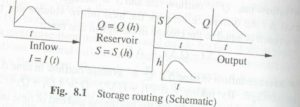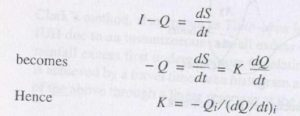# FLOOD ROUTING

FLOOD ROUTING – The stage and discharge hydrographs represent the passage of waves of river depth and discharge respectively.

As this wave moves down the river, the shape of the wave gets modified due to various factors, such as channel storage, resistance, lateral addition or withdrawal of flows, etc.

When a flood wave passes through a reservoir, its peak is attenuated and the time base is enlarged due to the effect of storage.

Flood waves passing down a river have their peaks attenuated due to friction if there is no lateral inflow.

The addition of lateral inflows can cause a reduction of attenuation or even amplification of a flood wave.

Flood routing is the technique of determining the flood hydrograph at a section of a river by utilizing the data of flood flow at one or more upstream sections.

The hydrologic analysis of problems such as flood forecasting, flood protection, reservoir design  and spill design  invariably include  flood routing.

In these applications  two broad categories of routing can be recognised.

These are:

Reservoir routing

Channel routing

In reservoir routing the effect of a flood wave entering a reservoir is studied. Knowing the  volume — elevation  characteristic  of  the  reservoir  and  the  outflow — elevation relationship for the spillways and other outlet structures in the reservoir, the effect of a flood wave entering the reservoir is studied to predict the! variations of reservoir elevation and outflow discharge with time.

This form of reservoir routing is essential (i) in the design of the capacity of spill and other) reservoir outlet structures and (ii) in the location and sizing of the capacity of reservoirs to meet specific requirements.

In channel routing  the change  in the  shape  of a hydrograph  as it travels  down a channel is studied.

By considering a channel reach and an input hydrograph at the upstream end, this form of routing aims to predict the flood hydrograph at various

sections of the reach. Information on the flood-peak attenuation and the duration of levels obtained by channel routing is of utmost importance in ‘ operations and flood- protection works.

A variety of routing methods are available and they can be broadly classified into two categories as: (i) hydrologic routing and (ii) hydraulic routing. 0 methods employ essentially the equation of continuity.

Hydraulic methods, on the other hand, employ the continuity equation  together with the equation of motion of unsteady flow-

The basic  differential  equations  used  in  the  hydraulic  routing,  known  as  St.Venant equations afford a better description of flow than hydrologic methods.

## HYDROLOGICAL STORAGE ROUTING (LEVELPOOL ROUTING)

A flood wave 1(t) enters a reservoir Provided with an outlet such as a spill Way T outflow is a function of the reservoir elevation only, i.e. Q =Q (h).

The Storage in the reservoir is a function of the reservoir elevation s = s(h)Further, due to the Passage of the flood wave through the reservoir, the water level in the reservoir changing with time h =h (t) and hence the storage and discharge change with time required to find the variation of s, h and Q with time.

where H = head over the spill way, L= effective length of the Spill way crest and C = coefficient of discharge .

Similarly for other forms of outlets such as gated Spill ways sluice gates, etc. other relations for Q (h) will be available,

For reservoir routing, the following data have to be known:

1. Storage volume vs elevation for the reservoir:

2. Water surface  elevation  vs  outflow  and  hence  storage    outflow discharge;

3 inflow hydrograph I = I(t and

4. Initial values of S,/and Q at time: =0

There are a V of methods available for routing of floods through a reservoir. All of them  use but in various  re arranged  manners.

As the  horizontal  surface  is assumed in the reservoir, the storage routing is also known as leve1 pool routing.

HYDRAULIC METHOD FOR FLOOD ROUTING

Only for highly simplified eases can one obtain the analytical solution of these equations. The development of modern, high-speed digital computers during the past two decades has given rise to the evolution of many indicated  numerical techniques.

The  various  numerical  methods  for  solving  St.venant  equations  can  be  broadly classified into two categories:

Approximate Methods

Complete Numerical methods

These methods are based on the equation of continuity only or on a drastically curtailed equation of motion.

The hydrological method of storage routing and Muskingum channel routing belong to this category. Other methods in this category are diffusion analogy and kinematic wave models.

Complete Numerical Methods

These are the essence of the hydraulic method of routing and are classified into many categories as below:

In the direct method, the partial derivatives are replaced by finite differences and the resulting algebraic equations are then solved. In the method of characteristics (MOC)

St Venant equations are converted into a pair of ordinary differential equations (i.e. characteristic  forms) and  then  solved  by finite  difference  techniques.

In the  finite element method (FEM) the system is divided into a number of elements and partial differential equations are integrated at the nodal points of the elements.

The numerical schemes are further classified into explicit and implicit methods.

In the explicit method the algebraic equations are linear and the dependent variables are extracted explicitly at the end of each time step.

In the implicit method the dependent Variables occur implicitly and the equations are nonlinear. Each of these two methods have a host of finite- differentiating schemes to choose from.

ROUTING IN CONCEPTUAL HYDROGRAPH DEVELOPMENT

Even though the routing of floods through a reservoir or channel discuss previous section  were  developed  for  field  use,  they  have  found  another  important  in  the conceptual studies of hydrographs.

The FLOOD ROUTING through a reservoir attenuation and channel  routing  which  gives translation  to an input hydrograph  are treated as two basic modifying operators.

The following two fictitious intensities in the studies for development of synthetic hydrographs through conceptual models.

1. Linear  reservoir:  a  reservoir  in  which  the  storage  is  directly  proportional  to discharge, (S = KQ). This element is used to provide attenuation to flood wave.

2. Linear channel: a fictitious channel  in which  the  time  required  to discharge  Q through a given reach is constant. An inflow hydrograph pass through such a channel with only translation and no attenuation.

Conceptual modelling for Hill development has undergone rapid progress Since the first work by Zoch (1937). Detailed reviews of various contributions to this field are available in Refs 2 and 3 and the details are beyond the scope of this book However, a

simple method, viz, Clark’ s method (1945) which utilizes the Muskingum method of routing through a linear reservoir is indicated below as a typical example of the use of routing in conceptual models.

Routing

The linear reservoir at the outlet is assumed to be described by S = KQ, where K is the storage time constant. The value of K can be estimated by considering the point of inflection P of a surface runoff hydrograph .

At this point the inflow into the channel has ceased and beyond  this point  the flow is entirely due to withdrawal  from the channel storage, The continuity equationwhere suffix i refers to the point of inflection, and K can be estimated from a known surface runoff hydrograph of the catchment

The constant K can also he estimated from the data on the recession limb of a hydrograph.

Knowing K of the linear reservoir, the inflows at various times are routed by the Muskingum  method.

Note  that  since  a  linear  reservoir  is used  .  The  inflow  rate between an inter-isochrone area A, km with a time interval   t (h) is Routing  of the time-area histogram by the above equation gives the ordinates of IUH for the catchment.

Using this IUH- any other D-h unit hydrograph can be derived.